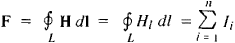# Magnetomotive Force

Also found in: Dictionary, Thesaurus, Acronyms, Wikipedia.

## magnetomotive force

[mag¦nēd·ō′mōd·iv ′fōrs]
(electromagnetism)
The work that would be required to carry a magnetic pole of unit strength once around a magnetic circuit. Abbreviated mmf.

## Magnetomotive Force

a quantity that characterizes the magnetic action of an electric current. The magnetomotive force is introduced in calculations of magnetic circuits by analogy with the electromotive force in electric circuits.

The magnetomotive force F is equal to the circulation of the vector of magnetic field intensity H through a closed circuit L that encompasses the electric currents generated by the magnetic field:(in the International System of Units). Here HI is the projection of H onto the direction of the element of the integration circuit d I and n is the number of conductors (coils) carrying a current Ii that are encompassed by the circuit. The unit of magnetomotive force is the ampere (or ampere-turn) in the International System of Units and the gilbert in the cgs system of units (symmetrical); 1 A = (4π/10) Gb ~ 1.2566 Gb.

References in periodicals archive ?
Analysis of the full pitch coil winding shows, that relative magnetomotive force [F.
2] = 1/2 are amplitudes of relative magnetomotive force components.
According to results from Table III, the space distribution of instantaneous rotating magnetomotive force in a defined moment of time was created (Fig.
For the analysed six-phase chain winding, the harmonic analysis equations of rotating magnetomotive force (Fig.
After constructing the instantaneous space distribution of the traveling magnetomotive force created by CLIM inductor the windings of which are connected using one of the unconventional methods (Fig.
i]--the asymmetry of i-th rectangle of the stair-shape half-period of magnetomotive force in respect of the selected coordinate axis, expressed in electrical degrees of the fundamental space harmonic; v - the number of odd space harmonic; v - the number of even space harmonic.
According to the results in Table 3 the spatial distribution of instantaneous rotating magnetomotive force in the set moment of time (t = 0) is determined (Fig 1, b).
1, b the parameters of positive half-period of instantaneous rotating magnetomotive force of the considered winding are the following: k = 5; [F.
Using (6), (17) and (18), we express magnetomotive force N[I.
i] width of the i-th rectangle of the stair-shaped magnetomotive force expressed in electrical degrees of the fundamental space harmonic; [upsilon]--number of harmonic.
2v']--conditional amplitude value of the rotating magnetomotive force of the v'-th harmonics of the two-layer tree-phase fractional winding with q = 3/2; [F.

Site: Follow: Share:
Open / Close